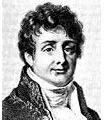# What is Fourier’s Law of Heat Conduction?

## Introduction

Heat conduction is transfer of heat from a warmer to a colder object by direct contact. A famous example is shown in A Christmas Story, where Ralphie dares his friend Flick to lick a frozen flagpole, and the latter subsequently gets his tongue stuck to it.

The mathematical model was first formulated by the French physicist Jean Baptiste Joseph Fourier, he of the eponymous Fourier Series. He found that heat flux is proportional to the magnitude of a temperature gradient. His equation is called Fourier’s Law.

## Fourier’s Law Of Heat ConductionIn a one dimensional differential form, Fourier’s Law is as follows:

1) q = Q/A = -kdT/dx.

The symbol q is the heat flux, which is the heat per unit area, and it is a vector. Q is the heat rate. dT/dx is the thermal gradient in the direction of the flow. The minus sign is to show that the flow of heat is from hotter to colder. If the temperature decreases with x, q will be positive and will flow in the direction of x. If the temperature increases with x, q will be negative, and will flow opposite to the direction of x. In the International System of Units SI, q is watts per meter squared (w/m2).

The constant k is the thermal conductivity, and is used to show that not all materials heat up or retain heat equally well. In SI units, k is W/m*K, where W is watts, m is meters, and K is kelvin. It may also be J/m*s*K, where J is joules and s is seconds. In the English system, it is Btu/h*ft*oF, or British thermal units per horsepower*foot*Fahrenheit.

The thermal conductivity is larger for conductors than insulators. Silver is an excellent conductor at 428 W/m*k, and so is copper with its value of 401 W/m*k. Air and wool are insulators and are poor conductors; they are 0.026 and 0.043 W/m*k, respectively.

The heat transfer or conduction rate is a scalar and is

2) Q = -kA ΔT/L,

where L is the length of the slab. ΔT is the temperature difference between two different surfaces.

Equations 1 and 2 show that heat can be considered to be a flow. The flow of heat depends upon the thickness of the material, the area, and the conductivity, all of which combine to retard or resist this flow.

## Example

A slab that is made from copper has a length of 10 cm and an area that is 90 cm2. The front side is heated to 150 oC and the back to 10 oC. Find the heat flux q and the heat flow rate Q in the slab once steady state is reached. Assume dT/dx is constant.

From equation 1, we have q = -kA (Tback – Tfront)/L = – (401 W/m*K) * (10 oC – 150 oC)/0.1 m = 5.6 x 10(5) W/m2. The heat rate is Q = qA = (90 x 10-4 m2) = 5.1 x 103 watts or J/s.

## References

Fundamentals of Physics by Halliday, Resnick, and Walker

A Heat Transfer Textbook by John H. Lienhard IV and John H. Lienhard V

Image Credits

Joseph Fourier courtesy of Commons.Wikimedia.org

## Resources

Joseph Fourier Biography

Elementary Heat Conduction